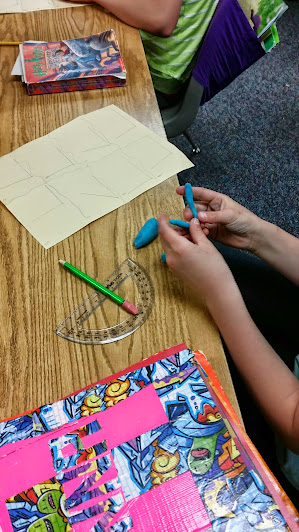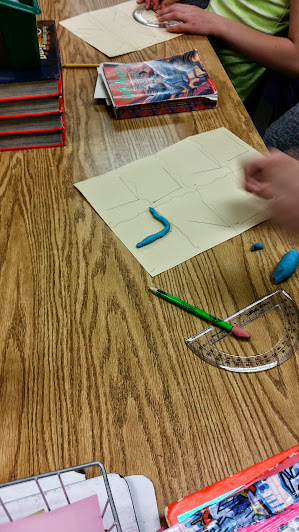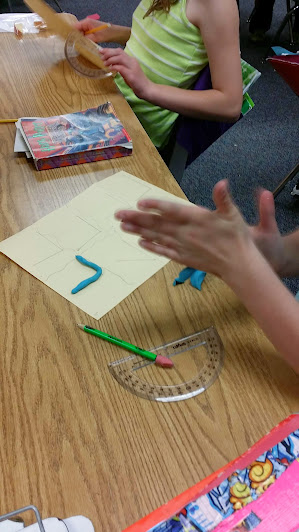# Drawing Angles

7 teachers like this lesson
Print Lesson

## Objective

SWBAT use a protractor to draw angles.

#### Big Idea

In this direct instruction lesson, students use a protractor to draw angles specified.

## Concept Development

50 minutes

This lesson is a direct instruction lesson.  In order for students to be successful in drawing angles accurately and precisely, they need practice and to be able to compare their angle to one this is drawn correctly.

I begin this lesson by showing students how to draw an angle using a protractor. My students have had prior lessons using a protractor to measure angles, but drawing angles is somewhat more challenging for them.

I show students how I draw angles using a protractor under my document camera. I tell them the steps like this:  Draw a vertex on the paper and extend a straight edge ray from it along the bottom. Place the protractor on the vertex and line up the base line with the ray you drew. Find the specified measure you are supposed to draw the angle to along the measures on the protractor and mark that spot with a small dot using your pencil. Lift the protractor and use the straight edge to connect the vertex to the dot.  Label the vertex by giving it a letter name and record the degree of measure for the angle.

Next, I give students various angle measurements (about 5) and ask them to draw angles. I write the measurements on the white board and I circulate the room and help as needed, making sure students use the protractor correctly.

Once it appears most students are proficient in this skill, I introduce the next activity - Guess My Angle.  In this activity, students fold their paper into 8 equal rectangles. In each rectangle they construct an angle and write the angle measurements on the back of their paper in the corresponding rectangle.  Each student must finish all eight angles before the rest of the activity can continue.

My students will need clay for a future lesson, so while they are waiting for everyone to finish, I let them "play with clay." They love this and stay quiet so all students can finish.

You can see in these photos, a student is making clay models of her angles as she waits for her partner to finish.The second part of the Guess My Angle activity involves students switching papers with their learning partners.   Students must measure each angle their partner drew (without looking at the answers on the back).  Partners measure the angle in each rectangle and then write the measurement in that rectangle. When all angles are measured, students switch back papers and "correct" their partners work.  Students then must have a partner conversation about any measurement that were marked incorrect and determine if the error was a measuring error or a drawing error.

In this video below, you can see a student using a protractor to measure the angle degree of the angles his partner created.

Listen in as this partner talks about their errors.